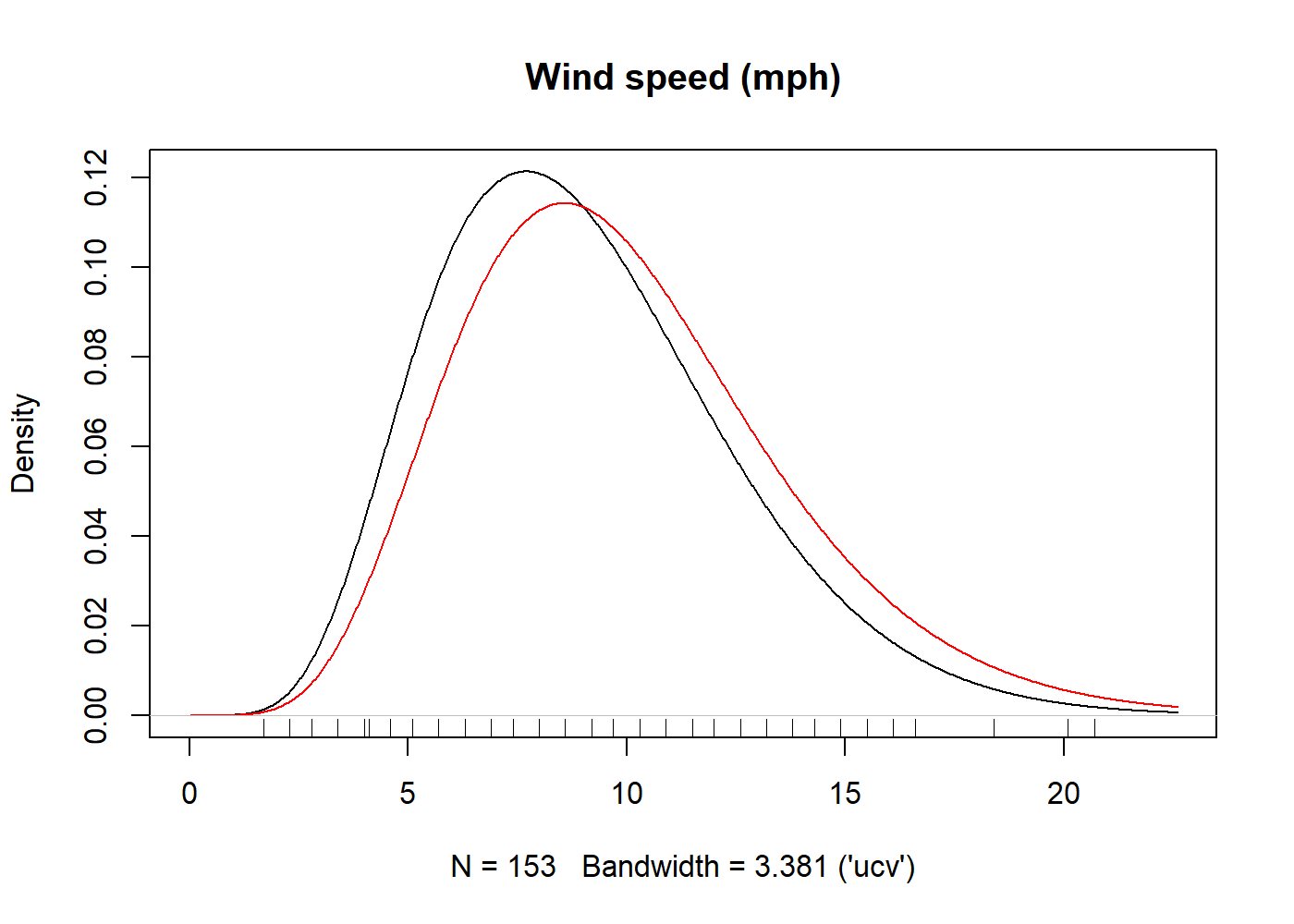# kdensityAn `R` package for univariate kernel density estimation with parametric starts and asymmetric kernels.

## Overview

kdensity is an implementation of univariate kernel density estimation with support for parametric starts and asymmetric kernels. Its main function is `kdensity`, which is has approximately the same syntax as `stats::density`. Its new functionality is:

• `kdensity` has built-in support for many parametric starts, such as `normal` and `gamma`, but you can also supply your own.
• It supports several asymmetric kernels ones such as `gcopula` and `gamma` kernels, but also the common symmetric ones. In addition, you can also supply your own kernels.
• A selection of choices for the bandwidth function `bw`, again including an option to specify your own.
• The returned value is callable: The density estimator returns a density function when called.

A reason to use `kdensity` is to avoid boundary bias when estimating densities on the unit interval or the positive half-line. Asymmetric kernels such as `gamma` and `gcopula` are designed for this purpose. The support for parametric starts allows you to easily use a method that is often superior to ordinary kernel density estimation.

## Installation

First you need to install the package `devtools` from `CRAN`. From inside `R`, use the following command.

``devtools::install_github("JonasMoss/kdensity")``

This installs the latest version of the package from GitHub. Call the `library` function and use it just like `stats:density`, but with optional additional arguments.

``````library("kdensity")
plot(kdensity(mtcars\$mpg, start = "normal"))``````

## Description

Kernel density estimation with a parametric start was introduced by Hjort and Glad in Nonparametric Density Estimation with a Parametric Start (1995). The idea is to start out with a parametric density before you do your kernel density estimation, so that your actual kernel density estimation will be a correction to the original parametric estimate. This is a good idea because the resulting estimator will be better than an ordinary kernel density estimator whenever the true density is close to your suggestion; and the estimator can be superior to the ordinary kernal density estimator even when the suggestion is pretty far off.

In addition to parametric starts, the package implements some asymmetric kernels. These kernels are useful when modelling data with sharp boundaries, such as data supported on the positive half-line or the unit interval. Currently we support the following asymmetric kernels:

These features can be combined to make asymmetric kernel densities estimators with parametric starts, see the example below. The package contains only one function, `kdensity`, in addition to the generics `plot`, `points`, `lines`, `summary`, and `print`.

## Usage

The function `kdensity` takes some `data`, a kernel `kernel` and a parametric start `start`. You can optionally specify the `support` parameter, which is used to find the normalizing constant.

The following example uses the data set plots both a gamma-kernel density estimate with a gamma start (black) and the the fully parametric gamma density. The underlying parameter estimates are always maximum likelood.

``````library("kdensity")
kde = kdensity(airquality\$Wind, start = "gamma", kernel = "gamma")
plot(kde, main = "Wind speed (mph)")
lines(kde, plot_start = TRUE, col = "red")
rug(airquality\$Wind)``````Since the return value of `kdensity` is a function, it is callable, as in:

``````kde(10)
#>  0.09980471``````

You can access the parameter estimates by using `coef`. You can also access the log likelihood (`logLik`), AIC and BIC of the parametric start distribution.

``````coef(kde)
#>     shape      rate
#> 7.1872898 0.7217954
logLik(kde)
#> 'log Lik.' 12.33787 (df=2)
AIC(kde)
#>  -20.67574``````